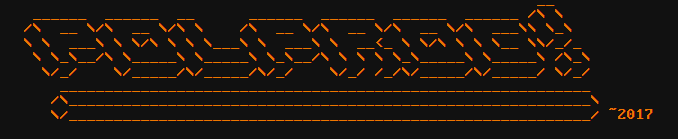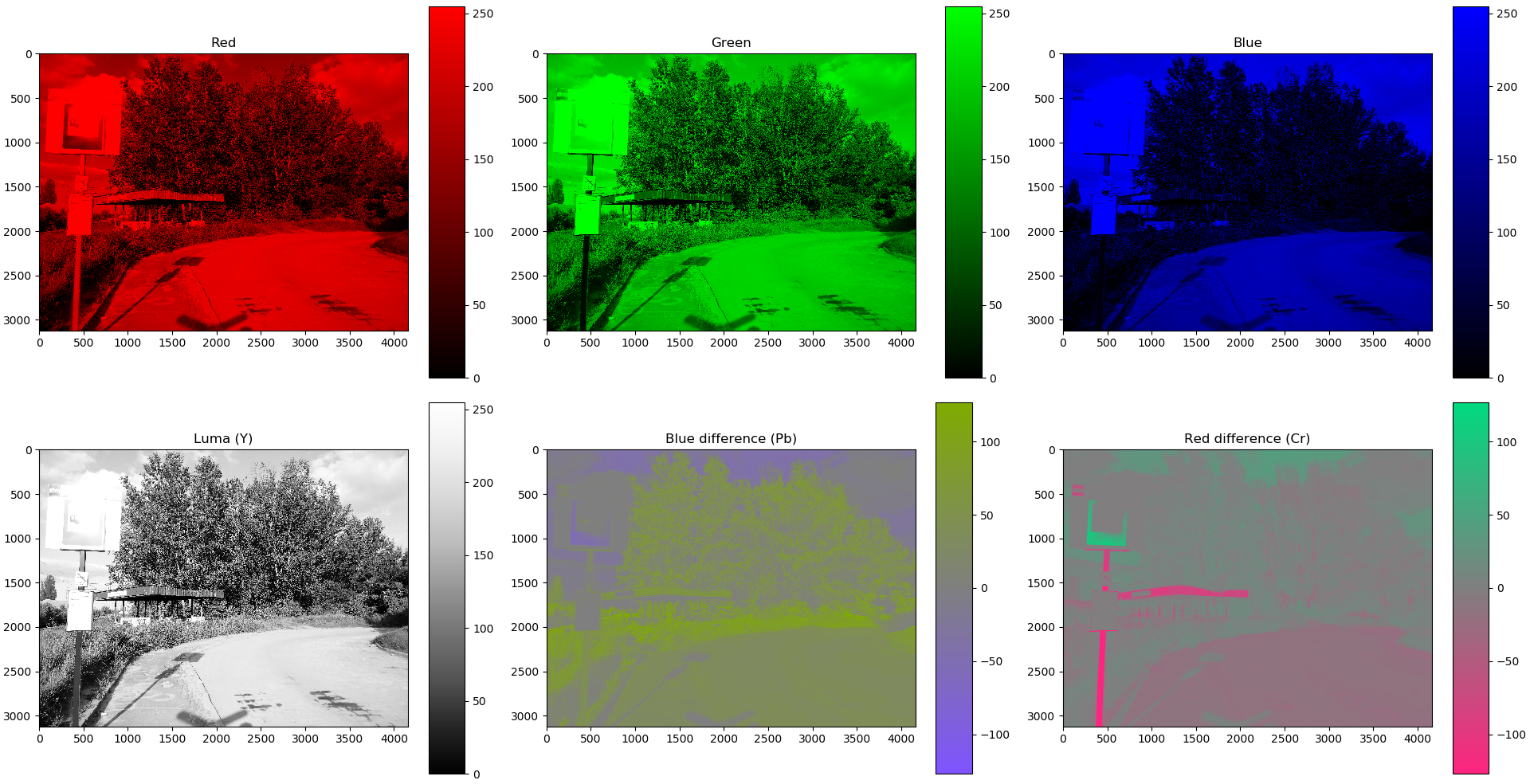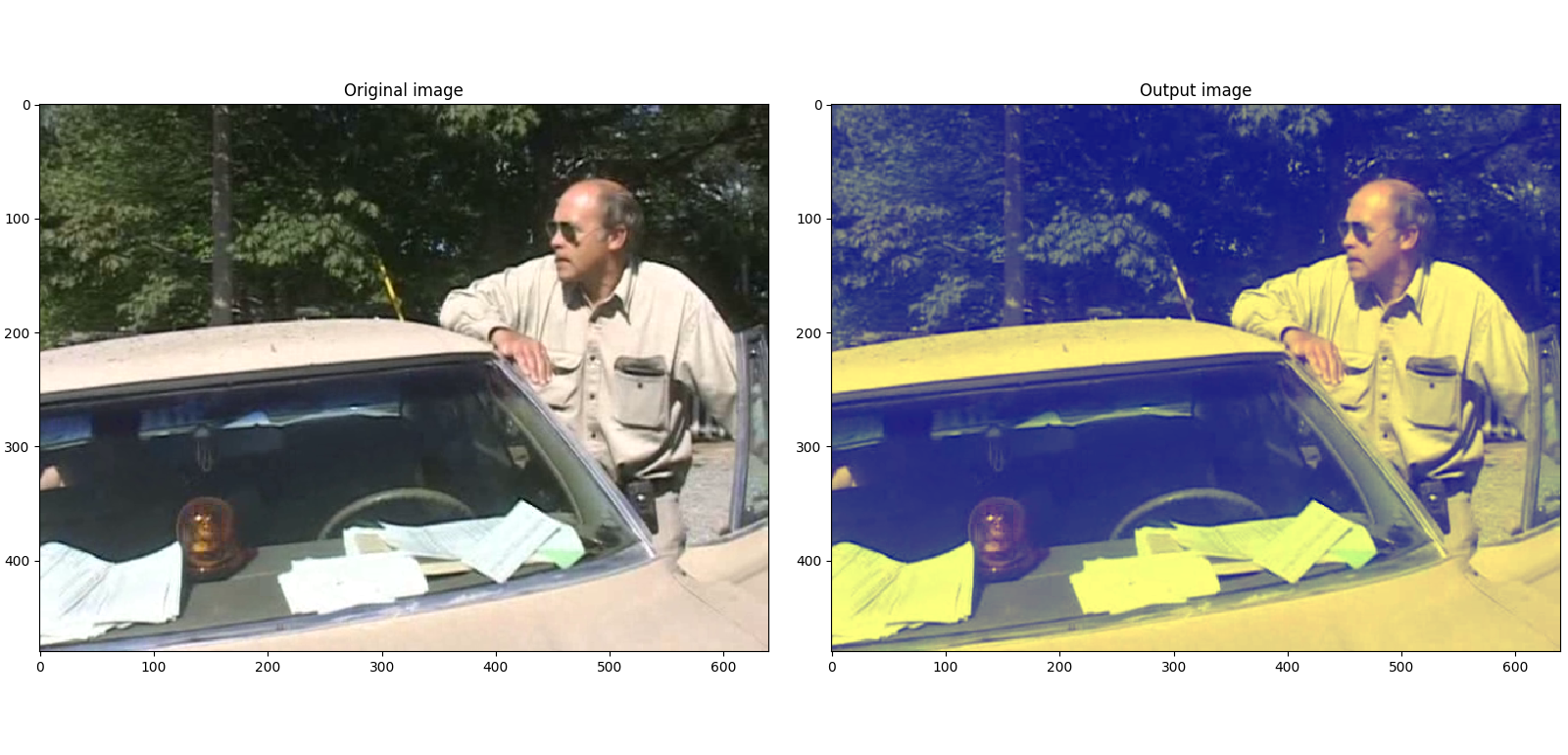# Elements of analogue images

### Deconstructing analogue video in python using numpy and image processing

Back to homepage

In this post we take a look at the quirks of the YCC color space, it's differences from the RGB model and what are the pros of using YCC over RGB in certain applications. We take apart several images to see what is chroma subsampling, and how much information each color channel carries in an image

## The components of composite video

Before we start analyzing images and the YCC color model, we need to undrstand how it is created, transmitted, and then recreated at the receiver

A composite video signal has to transmit all the information necesary to reproduce an image on the screen. It carries the brightness information, simply represented as a waveform between two chosen voltages (the black level and the white level). Timing signals such as horizontal and vertical sync, are represented as various blips below the black level, to the so called blank level. The signal is encoded line-by-line, so it only has vertical resolution.

Color information, in case of color video, is encoded by mixing the black and white signal with a high frequency sine wave. At each frame, a high frequency sine wave is added to the signal. The amplitude and phase of it carries two color channels.

Those three channels: brigtness - called Luma and denoted by Y, and red and blue color differences - denoted by Cb and Cr respectively, form the YCbCr color space.

By having the brightness and the two color channels, the receiver can convert the data to the RGB model, suitable for driving the red, green, and blue electron guns of a CRT, or the pixels on a color LCD. Since Pb and Pr were originally created by subtracting the red and blue channel from luma, reversing this operation gives the red and blue channel back. The green channel can then be calculated by subtracing R, and B from Y.

In the analogue realm, encoding the color information like that allowed black-and-white television sets to still receive and display the black-and-white part of the signal.

However the YCC color model is also widely used in the digital realm, and that's what Ill be discussing in this post...This is an example of how an RGB image is converted into YCbCr. I wrote the script some time ago in order to play with numpy and image processing. The script is available here.

## Deconstruction script

To be able to easily decompose an image to look at each channel separately we need a handy little script. Fortunately the numpy library for python does an extremely good job in making this simple. Let's take a look at the code that generates the image above

First of all we need some imports. This is trivial - imageio for loading the images as numpy arrays (you can also use matplotlib.image instead, but that only supports PNG files), matplotlib for plotting, os and sys for some general script matters

```import imageio
import matplotlib.pyplot as plt
import matplotlib.gridspec as gspc
from matplotlib.colors import LinearSegmentedColormap
import numpy as np
import os
import sys```

Then we load the image into memory

```if(len(sys.argv) < 2):
print("Usage: " + sys.argv + " <file>")
exit(1)

impath = sys.argv

Now that we have the imag array in the memory, we can start splitting it into R, G, and B channels. The array is 3-dimensional (the 3rd dimension being an array of 3 values - R, G and B)

```red = imag[:, :, 0]
grn = imag[:, :, 1]
blu = imag[:, :, 2]```

Now we can extract the Luma, Cb, and Cr channels from the image

```COEF_R = 0.2126
COEF_G = 0.7152
COEF_B = 0.0722

luma = imag[:, :, 0] * COEF_R + imag[:, :, 1] * COEF_G + imag[:, :, 2] * COEF_B

pb = luma - blu
pr = luma - red```

First we define the Rec. 709 coefficients for converting RGB to luma. Then we multiply each chanel by it's coefficient and sum them to get the luminance.

Pb and Pr channels are calculated by subtracting blue and red from the luma channel.

## Information density of RGB (Rec. 709)

Let's take a break here and look at the Rec. 709 coefficients for a moment. Notice how the green coefficient is about 10 times bigger than the blue one. That's because human eyes are the most sensitive to green and the least sensitive to blue, and that's also why the green channel carries stunning 70% of the luminance information.Furthermore, if you look at the above image, you will notice that the green channel is probably the clearest, of all the three. The blue image is barely recognizable, and the red is in betweenThe image above has had its blue channel all set to 128. As you can see it's prefectly acceptable, even though the blue information was discarded. This is how you create a trendy instagram filter ;) Looks like old fashioned colour slide film.Another image with the blue information discarded

todo

Back to homepage